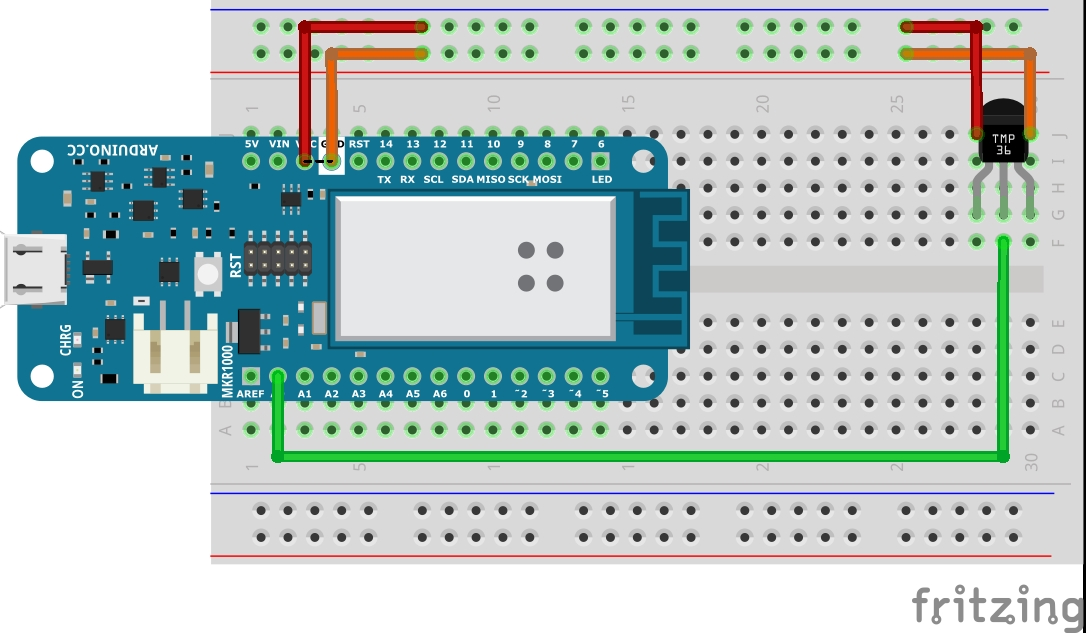Project tutorial# Sensing the temperature (S1.1) © GPL3+

In this hands on lab you will build a simple circuit to calculate the temperature.

• 338 views
• 0 respects

## Apps and online services

### Introduction:

In this hands on lab, you will build a temperature sensing device using the Arduino MKR1000.

This is the first in a series of hands on labs in the SMART Cities Program and introduces the basic concept of sensing the temperature.

### Putting it together:

There are five parts to this tutorial:

• Installing the Arduino IDE
• Assembling the circuit
• Viewing the results

### Part 1 - Installing the Arduino IDE

A quick tutorial for installing the plugin can be found Here

### Part 2 - Assembling the circuit

Using the below wiring diagram as a reference assemble your device

Note:

• The temperature sensor (TMP36) is connected to pin A0
• When inserting the Temperature sensor make sure that the flat side is facing towards you.

Now that you have a completed circuit you can load and run the sketch.

### Part 4 - Viewing the results

Having completed the programming of your device, now lets see the results by opening the serial monitor.

### The Final Result

On completion the device will display the temperature in the Serial Monitor.

## Code

##### Base CodeArduino
```// Temperature Sensor
int sensorPin = A0;   // Sets A0 as the input pin for the sensor
int sensorValue = 0;  // initialise a variable to store the value coming from the sensor
int Dres = 1024;      // set max 10-bit digital value
int VCC = 3300;       // set VCC voltage milliVolts
int offset = 500;        // set tolerance of 500 milliVolts
int scaling = 100;         // set the voltage scaling at 10mM / deg C
float temperature = 0.0; // set the initial temperature as 0
float voltage = 0.0;     // set the initial voltage to 0

void setup() {
Serial.begin(9600);
}

void loop() {

// read the value from the sensor:
Serial.print("sensorValue = ");
Serial.print(sensorValue);

// Calculate the voltage
voltage = sensorValue * (VCC/Dres); // milliVolts
Serial.print(" voltage = ");
Serial.print(voltage);
Serial.print(" VCC = ");
Serial.print(VCC);

// Calculate the temperature
temperature = (voltage - offset ) / scaling;
Serial.print(" temperature(C) = ");
Serial.println(temperature);

delay(1000);
}
```

## Schematics#### Author

• Paynter Technology

#### Published on

August 30, 2020
See similar projects
you might like

#### An IoT controlled temperature monitor (S1.3)

Project tutorial by greg

• 2,257 views
• 1 comment
• 1 respect

#### Portable Temperature Station

Project tutorial by Isaac100

• 13,940 views
• 42 respects

• 3,733 views
• 1 comment
• 13 respects

#### Temperature and Humidity Data Logger

Project tutorial by Wimpie van den Berg

• 43,275 views
• 37 respects

#### Temperature Monitoring with Arduino MKR1000 and ARTIK Cloud

Project tutorial by FoxLab

• 13,234 views